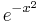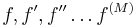# Informatics1-2018/HW6

## 1. Exercise

3 points

The Collatz or 3n+1 problem is the following:

• Let n be a positive integer.
• Let g(n) = n/2 is n is even and 3n+1 is odd.
• Then one can iterate g over-and-over for any given number. For example starting with 98:
```{98, 49, 148, 74, 37, 112, 56, 28, 14, 7, 22, 11, 34, 17, 52, 26, 13, 40, 20, 10, 5, 16, 8, 4, 2, 1}
```

It is an interesting and unproven conjecture that starting from any number, you will reach 1 sooner or later. In the example above it took 25 steps. For example 5 -> 16 -> 8 -> 4 -> 2 -> 1 takes 5 steps.

Plot the number of steps needed to reach one against n, use ListPlot and n=1...1000

## 2. Exercise

### a)

3 points

Define a function T with three arguments:

• f a function
• n a natural number
• x0 a real number

For this values calculate the nth Taylor polynomial of f around x0.

For example:

```In:=  T[Exp,4,0]
Out:= 1 + x + x^2/2 + x^3/6 + x^4/24
```

Note that there is a buit-on function Series which does exactly this, but don't use that, implement it on your own! Use the sum symbol from the paletta and the Derivative.

### b)

3 points

Plot the function$e^{-x^2}$ and its derivatives on a single Plot. For a given M, plot$f, f', f'' \ldots f^{(M)}$ on the interval [ − 2,2] (this is M+1 functions in total). Use Manipulate to set the value of M.

# Handing in

```A1_borbely_HW6.nb